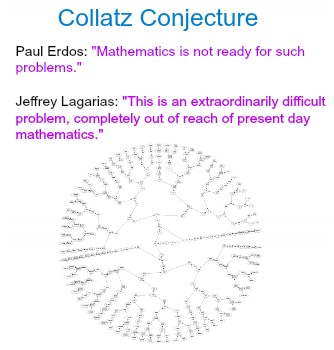Home Mathematics Collatz Conjecture: A problem for which mathematics is not ready

# Collatz Conjecture: A problem for which mathematics is not ready

Last updated on July 13th, 2020 at 10:16 pm

The beauty of mathematics is the number of mysteries the numbers hold themselves. Numbers are something which we are aware of since our pre-schooling days, isn’t it? But, these numbers have also given many sleepless nights to many mathematicians.

So, what makes these simple numbers so complex? The answer will be the properties they possess.

For example, the perfect number- In number theory, a perfect number is a positive integer that is equal to the sum of its proper positive divisors, that is, the sum of its positive divisors excluding the number itself. Example, 6 has factors- 1,2 & 3, also their sum, 1+2+3 = 6.

One such problem is the Collatz Conjecture proposed by mathematician Lothar Collatz.

## The Collatz Conjecture

Let’s play a little game. Create a sequence, or list, of numbers using the following rules:

2. If the integer is even, divide it by 2 to get the next number in the sequence (a1 / 2). If the integer is odd, multiply it by 3 and add 1 to the result (3a1 + 1) to get the next number in the sequence.
3. Repeat for each term.

For example, consider starting with the integer 3. Since 3 is odd, we get the next term in the sequence by multiplying it by 3 and adding 1.

• a2 = 3(3) + 1 = 10

Since 10 is even, we get the next term by dividing by 2.

• a3 = 10/2 = 5

5 is odd, so we get the next term by multiplying it by 3 and adding 1.

• a4 = 3(5) + 1 = 16

Continuing this pattern gives the following sequence:

• 3, 10, 5, 16, 8, 4, 2, 1,…

A few other examples starting at different integers are as follows:

• 4, 2, 1,…
• 5, 16, 8, 4, 2, 1,…
• 6, 3, 10, 5, 16, 8, 4, 2, 1,…

Do you notice anything that seems to happen in all of these types of sequences? Look closely and you may realize that all these series eventually arrive at 1! In mathematics, the fact that this specific type of sequence always appears to eventually arrive at 1 (regardless of the starting integer) is called the Collatz conjecture.## History of the Collatz Conjecture

Notice that the Collatz conjecture is called a conjecture, not a theorem. That’s because it has yet to be proven! The problem of proving the Collatz conjecture is also called the 3x + 1 problem and has stumped mathematicians worldwide for many years.

The conjecture itself got its name from Lothar Collatz due to his research and work on the problem. That being said, Collatz does not claim the origin of the problem itself, even though many say that he first proposed it in 1937. However, again, there is no concrete evidence that this is the case.

Part of the reason that the problem’s origin is a bit sketchy is that of its difficulty. No one could produce any important results when working with the problem, so nothing was published about it until 1971. At any rate, it has kept mathematicians mystified for over 60 years since it has yet to be proven!

Proving the conjecture is said to be one of the most difficult problems in mathematics. Paul Erdos, a well-known Hungarian mathematician, once said that ”Mathematics is not ready for such problems” when discussing the conjecture and its proof. Even as recent as 2010, Jeffrey Lagarias, a mathematics professor at the University of Michigan, stated that based on what we know about the problem of proving this conjecture, ”This is an extraordinarily difficult problem, completely out of reach of present-day mathematics.’

Video Courtesy- “Numberphile

Besides this, you can view our video and blog collections in the Video Section & Blog Section of the website.http://scilynk.in
Abhijeet is a 4th-year Undergraduate Student at IIT Kharagpur. His major inclination is towards exploring the science behind the things of our day-to-day life.

### Chandrayaan 3 Mission: India’s Next Step in Lunar Exploration

Chandrayaan 3 is India's next lunar mission, following the success of Chandrayaan 2 in 2019. The mission is set to launch next...

### Nobel Memorial Prize in Economic Sciences 2022 | US Trio Bags the Award

Three economists from the United States claim the prestigious award in Economics this year. Ben Bernanke, Douglas Diamond and Philip Dybvig share...

### Nobel Prize In Chemistry 2022 | Sharpless For The Second Time

Carolyn R. Bertozzi, Morten Meldal and K. Barry Sharpless become the 2022 Chemistry Nobel Laureates. They equally share the Nobel Prize in...

### Nobel Prize Winner Svante Paabo | Nobel Prize In Physiology or Medicine 2022

A phalanx (finger bone) excavated from the dig sites of Denisova caves in Siberia was found to have the potential to tell...